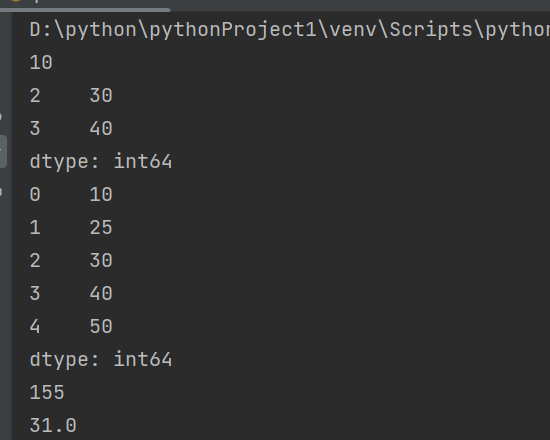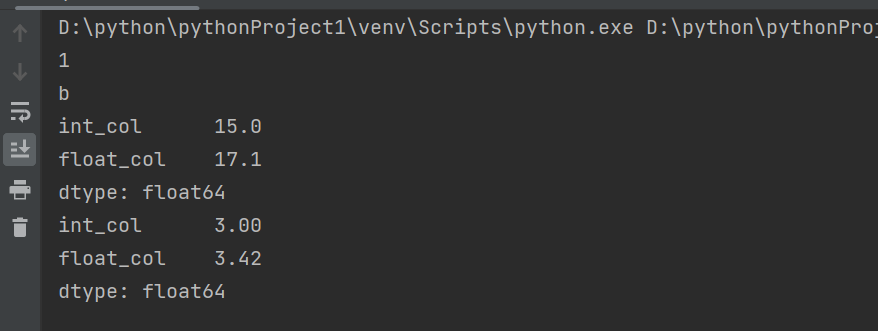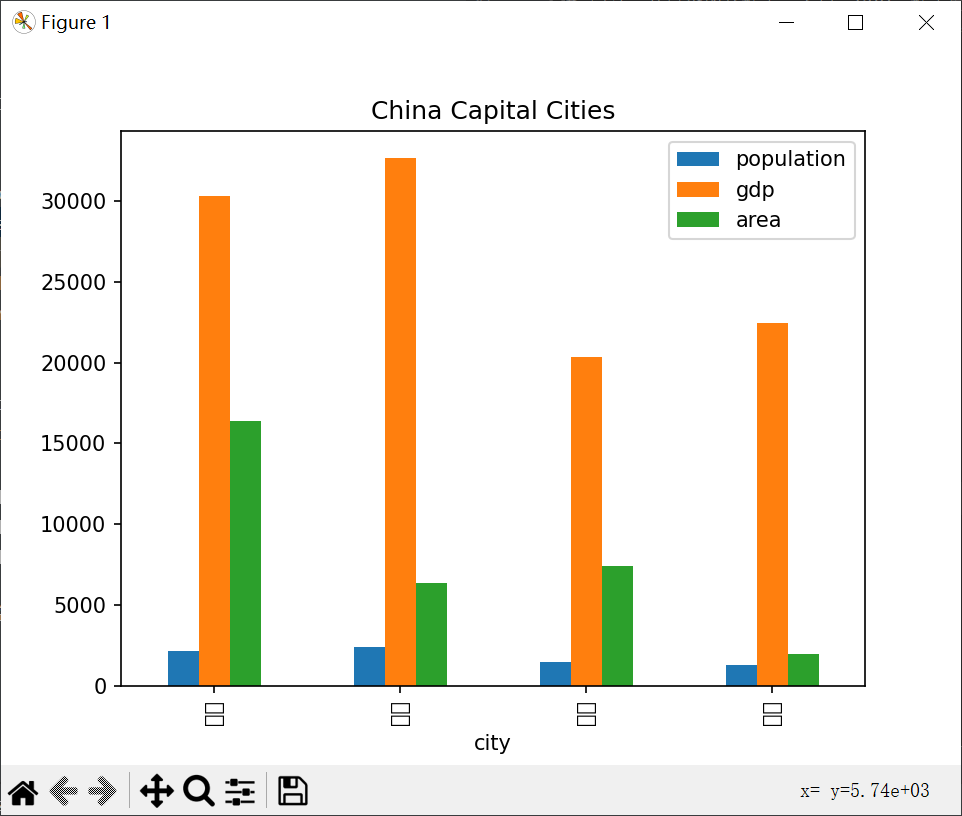# 2023（春）Python程序设计作业5：Pandas基础技能及综合应用

## 作业要求

1.    [基础要求]  基于Jupyter Notebook 完成以下实验一、实验二、实验三；
2.    [重点要求]  修改以下示例代码，以测试不同知识点。在博客上写出你：
•  修改的代码、
•  修改的愿意（意图）
•  代码运行的结果
•  你的结论

## 实验一：Series对象的应用

### 实验要求：

• 定义一个Series对象，包含5个整数数据；
• 访问、修改Series对象中的数据；
• 打印Series对象；
• 对Series对象进行计算，如求和、求平均值等。
``````import pandas as pd

# 定义一个Series对象
s = pd.Series([10, 20, 30, 40, 50])

# 访问Series对象中的数据
print(s)    # 输出第一个元素
print(s[2:4])  # 输出第3个到第4个元素

# 修改Series对象中的数据
s = 25

# 打印Series对象
print(s)

# 对Series对象进行计算
print(s.sum())  # 求和
print(s.mean())  # 求平均值``````## 实验二：DataFrame对象的应用

### 实验要求：

• 定义一个DataFrame对象，包含3个列，每列分别为整数、浮点数和字符串类型；
• 访问、修改DataFrame对象中的数据；
• 对DataFrame对象进行计算，如求和、求平均值等。
``````# 定义一个DataFrame对象
data = {'int_col': [1, 2, 3, 4, 5], 'float_col': [1.2, 2.3, 3.4, 4.5, 5.6], 'str_col': ['a', 'b', 'c', 'd', 'e']}
df = pd.DataFrame(data)

# 访问DataFrame对象中的数据
print(df['int_col'])  # 输出第一行第一列的数据
print(df.loc[1, 'str_col'])  # 输出第二行第三列的数据

# 修改DataFrame对象中的数据
df.loc[2, 'float_col'] = 3.5

# 对DataFrame对象进行计算
numeric_cols = df.select_dtypes(include=['int64', 'float64']).columns
print(df[numeric_cols].sum())  # 求和
print(df[numeric_cols].mean())  # 求平均值``````## 实验三：综合实例

### 实验要求：

• 定义一个包含省会城市、人口、GDP、城市面积的DataFrame对象；
• 计算各种排名，如人口最多的城市、GDP最高的城市等；
• 使用Pandas绘图，可视化上述实验结果。
``````import pandas as pd
import matplotlib.pyplot as plt

# 定义一个包含省会城市、人口、GDP、城市面积的DataFrame对象
data = {'city': ['北京', '上海', '广州', '深圳'], 'population': [2171, 2424, 1500, 1303],
'gdp': [30320, 32679, 20353, 22458], 'area': [16410, 6340, 7434, 1996]}
df = pd.DataFrame(data)

# 计算各种排名
pop_rank = df['population'].rank(ascending=False)
gdp_rank = df['gdp'].rank(ascending=False)
area_rank = df['area'].rank(ascending=False)

# 将排名添加到DataFrame对象中
df['pop_rank'] = pop_rank
df['gdp_rank'] = gdp_rank
df['area_rank'] = area_rank

# 使用Pandas绘图，可视化实验结果
df.plot(kind='bar', x='city', y=['population', 'gdp', 'area'], title='China Capital Cities')
plt.show()``````

修改意图：
- 将省会城市的人口、GDP和城市面积修改为不同的值。
- 计算新的排名，包括人口最多的城市、GDP最高的城市和城市面积最大的城市。
- 使用条形图可视化实验结果。...全文
5 回复 打赏 收藏 转发到动态 举报极简python入门课程
1、课程基于Python3讲解，从基础技能到实际案例，并辅以练习演示、课后作业及社区答疑；2、带你充分建立编程思维，扎实编码能力，学习后可独立完成小型程序开发；3、学会多线程等高级编程方法，编写出复杂的 Python 应用；4、通过标准库和第三方库，编写出能解决实际问题的工具；5、完整的 Python 入门+实战学习路径。2023Python程序设计作业1： 基础语法之控制流程

159发帖与我相关我的任务
python 高校 江苏省·南通市• 近7日
• 近30日
• 至今